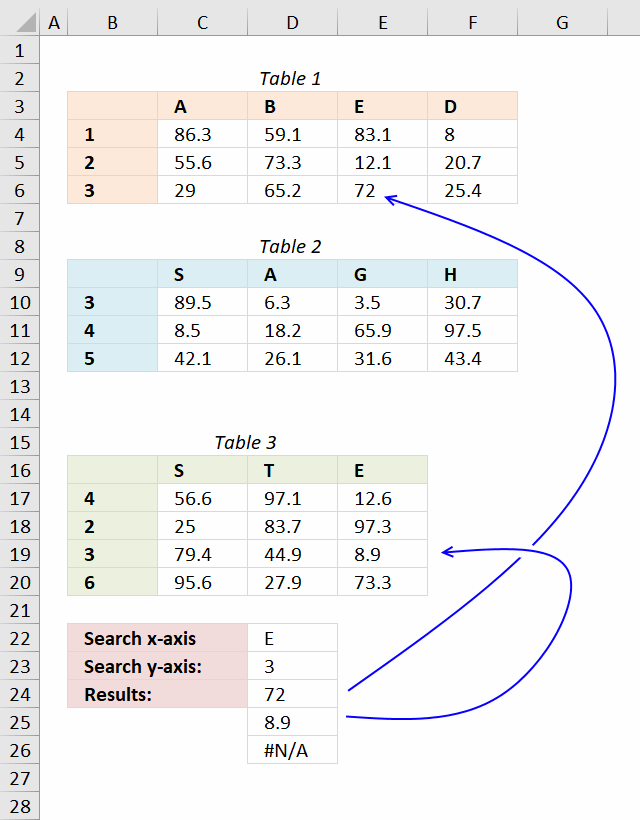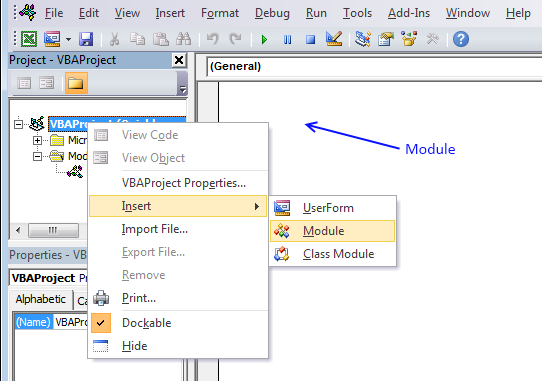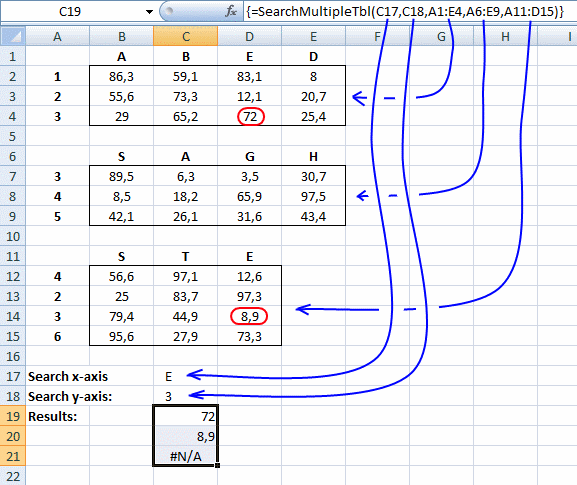Author: Oscar Cronquist Article last updated on March 16, 2019This article describes a User Defined Function that lookups values in multiple cross reference tables based on two conditions. A User defined function is a custom function anyone can use, simply copy the VBA code and paste to your workbooks code module and you are good to go.

The custom function demonstrated in this article accepts an arbitrary number of range arguments (table ranges). The custom function returns the first match in each table if there is a match.

Array formula in cell C24:C26:

=SearchMultipleTbl(D22,D23,B3:F6,B9:F12,B16:E20)

How to enter an array formula

1. Select cell range C24:C26
2. Copy above formula
3. Paste formula
4. Press and hold Ctrl + Shift
5. Press Enter once
6. Release all keys

### User Defined Function Syntax

SEARCHMULTIPLETBL(xaxis, yaxis, cellrange1, [cellrange2])

### Arguments

 Parameter Text xaxis Required. The x-axis condition you want to search for. yaxis Required. The y-axis condition you want to search for. cellrange1 Required. The range you want to add. [cellrange2] Optional. You may have up to 255 additional argument cell ranges.

VBA Code:

```'Name function and arguments
Function SEARCHMULTIPLETBL(xaxis As Variant, yaxis As Variant, ParamArray cellranges() As Variant)
'Declare variables and data types
Dim i As Integer, x As Variant, y As Variant
Dim temp() As Variant, xrange As Range, yrange As Range
'Redimension array variable temp so it may grow
ReDim temp(0)

'Iterate through all cell ranges
For i = LBound(cellranges) To UBound(cellranges)
'Enable error handling
On Error Resume Next
'Save first row to xrange object
Set xrange = cellranges(i).Rows(1)
'Save first column to yrange object
Set yrange = cellranges(i).Columns(1)
'Find position of matching value in row and column
x = Application.WorksheetFunction.Match(xaxis, xrange, 0)
y = Application.WorksheetFunction.Match(yaxis, yrange, 0)
'If found an error is not returned and Err.Number becomes 0 (zero)
If Err.Number = 0 Then
temp(UBound(temp)) = cellranges(i).Rows(y).Columns(x).Value
ReDim Preserve temp(UBound(temp) + 1)
Else
'Disable error handling
On Error GoTo 0
End If
'Continue with next cell range
Next i
'Remove last container in array
ReDim Preserve temp(UBound(temp) - 1)
'Return values in temp array rearranged (transposed)
SEARCHMULTIPLETBL= Application.Transpose(temp)

End Function
```

Where to copy vba code?1. Press Alt-F11 to open the Visual Basic Editor
2. Click Module on the Insert menu
3. Copy and paste vba code.
4. Exit visual basic editor